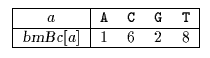Next: Quick Search algorithm Up: ESMAJ Prev: Reverse Colussi algorithm

# Horspool algorithm

Main features
• simplification of the Boyer-Moore algorithm;
• easy to implement;
• preprocessing phase in O(m+) time and O() space complexity;
• searching phase in O(mn) time complexity;
• the average number of comparisons for one text character is between 1/and 2/(+1).
Description

The bad-character shift used in the Boyer-Moore algorithm (see chapter Boyer-Moore algorithm) is not very efficient for small alphabets, but when the alphabet is large compared with the length of the pattern, as it is often the case with the ASCII table and ordinary searches made under a text editor, it becomes very useful.
Using it alone produces a very efficient algorithm in practice. Horspool proposed to use only the bad-character shift of the rightmost character of the window to compute the shifts in the Boyer-Moore algorithm.

The preprocessing phase is in O(m+) time and O() space complexity.

The searching phase has a quadratic worst case but it can be proved that the average number of comparisons for one text character is between 1/and 2/(+1).

The C code

The function preBmBc is given chapter Boyer-Moore algorithm.

```void HORSPOOL(char *x, int m, char *y, int n) {
int j, bmBc[ASIZE];
char c;

/* Preprocessing */
preBmBc(x, m, bmBc);

/* Searching */
j = 0;
while (j <= n - m) {
c = y[j + m - 1];
if (x[m - 1] == c && memcmp(x, y + j, m - 1) == 0)
OUTPUT(j);
j += bmBc[c];
}
}

```
The example

Preprocessing phasebmBc table used by Horspool algorithm

Searching phase

References
• AHO, A.V., 1990, Algorithms for finding patterns in strings. in Handbook of Theoretical Computer Science, Volume A, Algorithms and complexity, J. van Leeuwen ed., Chapter 5, pp 255-300, Elsevier, Amsterdam.
• BAEZA-YATES, R.A., RÉGNIER, M., 1992, Average running time of the Boyer-Moore-Horspool algorithm, Theoretical Computer Science 92(1):19-31.
• BEAUQUIER, D., BERSTEL, J., CHRÉTIENNE, P., 1992, Éléments d'algorithmique, Chapter 10, pp 337-377, Masson, Paris.
• CROCHEMORE, M., HANCART, C., 1999, Pattern Matching in Strings, in Algorithms and Theory of Computation Handbook, M.J. Atallah ed., Chapter 11, pp 11-1--11-28, CRC Press Inc., Boca Raton, FL.
• HANCART, C., 1993. Analyse exacte et en moyenne d'algorithmes de recherche d'un motif dans un texte, Ph. D. Thesis, University Paris 7, France.
• HORSPOOL R.N., 1980, Practical fast searching in strings, Software - Practice & Experience, 10(6):501-506.
• LECROQ, T., 1995, Experimental results on string matching algorithms, Software - Practice & Experience 25(7):727-765.
• STEPHEN, G.A., 1994, String Searching Algorithms, World Scientific.Next: Quick Search algorithm Up: ESMAJ Prev: Reverse Colussi algorithm

e-mails: {Christian.Charras, Thierry.Lecroq }@laposte.net
Tue Jan 14 15:03:31 MET 1997